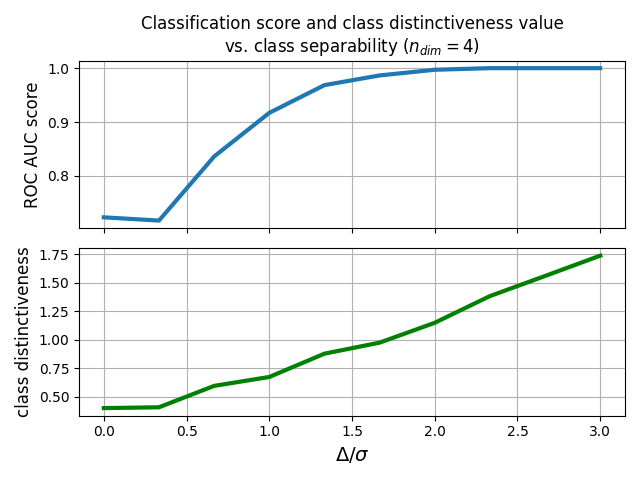# Classification accuracy vs class distinctiveness vs class separability¶

Generate several datasets containing data points from two-classes. Each class is generated with a Riemannian Gaussian distribution centered at the class mean and with the same dispersion sigma. The distance between the class means is parametrized by Delta, which we make vary between zero and 5*sigma. We illustrate how the accuracy of the MDM classifier and the value of the class distinctiveness  vary when Delta increases.

# Authors: Pedro Rodrigues <pedro.rodrigues@melix.org>
#          Maria Sayu Yamamoto <maria-sayu.yamamoto@universite-paris-saclay.fr>
#

import numpy as np
import matplotlib.pyplot as plt
from sklearn.model_selection import cross_val_score, StratifiedKFold

from pyriemann.classification import MDM
from pyriemann.datasets import make_gaussian_blobs
from pyriemann.classification import class_distinctiveness


Set general parameters for the illustrations

n_matrices = 100  # how many matrices to sample on each class
n_dim = 4  # dimensionality of the data points
sigma = 1.0  # dispersion of the Gaussian distributions
random_state = 42  # ensure reproducibility


Loop over different levels of separability between the classes

scores_array = []
class_dis_array = []
deltas_array = np.linspace(0, 3*sigma, 10)

for delta in deltas_array:
# generate data points for a classification problem
X, y = make_gaussian_blobs(n_matrices=n_matrices,
n_dim=n_dim,
class_sep=delta,
class_disp=sigma,
random_state=random_state,
n_jobs=4)

# measure class distinctiveness of training data for each split
skf = StratifiedKFold(n_splits=5)
all_class_dis = []
for train_ind, _ in skf.split(X, y):
class_dis = class_distinctiveness(X[train_ind], y[train_ind],
exponent=1, metric='riemann',
return_num_denom=False)
all_class_dis.append(class_dis)

# average class distinctiveness across splits
mean_class_dis = np.mean(all_class_dis)
class_dis_array.append(mean_class_dis)

# Now let's train a MDM classifier and measure its performance
clf = MDM()

# get the classification score for this setup
scores_array.append(
cross_val_score(clf, X, y, cv=skf, scoring='roc_auc').mean())

scores_array = np.array(scores_array)
class_dis_array = np.array(class_dis_array)


Plot the results

fig, (ax1, ax2) = plt.subplots(sharex=True, nrows=2)

ax1.plot(deltas_array, scores_array, lw=3.0, label=r'ROC AUC score')
ax2.plot(deltas_array, class_dis_array, lw=3.0, color='g',
label='Class Distinctiveness')

ax2.set_xlabel(r'$\Delta/\sigma$', fontsize=14)
ax1.set_ylabel(r'ROC AUC score', fontsize=12)
ax2.set_ylabel(r'class distinctiveness', fontsize=12)
ax1.set_title('Classification score and class distinctiveness value\n'
r'vs. class separability ($n_{dim} = 4$)',
fontsize=12)

ax1.grid(True)
ax2.grid(True)
fig.tight_layout()
plt.show()